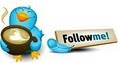#Compare one column with another using in a calculated field formula• ### Question

•Hi,

This calculated field formula computes the Distinct session conducted in every fiscal year

```=CALCULATE([Distinct sessions]
,DATESBETWEEN(Calendar1[DateKey]
,STARTOFYEAR(LASTDATE(Calendar1[DateKey]),"3-31")
,endOFYEAR(LASTDATE(Calendar1[DateKey]),"3-31")
)
)```

I now want to compute the Distinct session conducted in every fiscal year where one column is equal to another column

```=CALCULATE([Distinct sessions],Feedback[Attendees from]=Feedback[Organised by]
,DATESBETWEEN(Calendar1[DateKey]
,STARTOFYEAR(LASTDATE(Calendar1[DateKey]),"3-31")
,endOFYEAR(LASTDATE(Calendar1[DateKey]),"3-31")
)
)```

When I clicked on Check formula, I got this error

Calculation error in measure 'Feedback'[Fiscal Year Distinct sessions conducted independently]: The expression contains multiple columns, but only a single column can be used in a True/False expression that is used as a table filter expression.

Regards, Ashish Mathur Microsoft Excel MVP www.ashishmathur.com

Friday, February 22, 2013 2:44 PM

### All replies

•Just wondering if this works

```=CALCULATE([Distinct sessions],filter(Feedback, Feedback[Attendees from]=Feedback[Organised by])
,DATESBETWEEN(Calendar1[DateKey]
,STARTOFYEAR(LASTDATE(Calendar1[DateKey]),"3-31")
,endOFYEAR(LASTDATE(Calendar1[DateKey]),"3-31")
)
)```

If not, can you pass the file with some sample data? (Upload it in skydrive and pass the link?)

Cheers,
Jason | www.SqlJason.com• Marked as answer by Saturday, February 23, 2013 7:40 AM
Friday, February 22, 2013 6:20 PM
•Hi,

Thank you for your help.  Your formula did not yield any error but the result was still wrong.  I got the correct answer via these two formulas

```=CALCULATE([Distinct independent sessions]
,DATESBETWEEN(Calendar1[DateKey]
,STARTOFYEAR(LASTDATE(Calendar1[DateKey]),"3-31")
,endOFYEAR(LASTDATE(Calendar1[DateKey]),"3-31")
)
)```

I computed Distinct independent sessions via this formula

`=CALCULATE(DISTINCTCOUNT(Feedback[Date]),filter(Feedback,[Attendees from]=[Organised by]))`

Regards, Ashish Mathur Microsoft Excel MVP www.ashishmathur.com

Saturday, February 23, 2013 7:40 AM